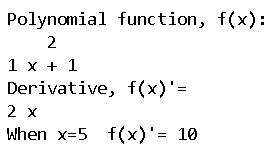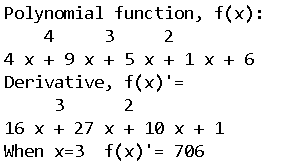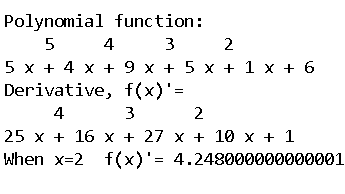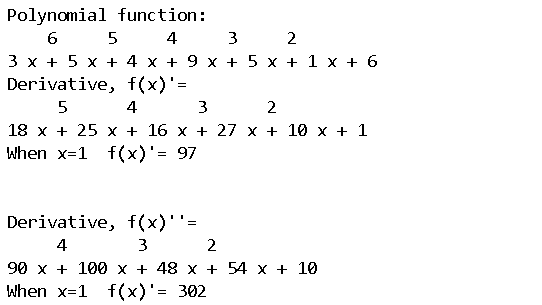# How to compute derivative using Numpy?

In this article, we will learn how to compute derivatives using NumPy. Generally, NumPy does not provide any robust function to compute the derivatives of different polynomials. However, NumPy can compute the special cases of one-dimensional polynomials using the functions numpy.poly1d() and deriv().

### Functions used:

• poly1d(): It helps to define a polynomial expression or a function.
• deriv(): Calculates and gives us the derivative expression

### Approach:

• At first, we need to define a polynomial function using the numpy.poly1d() function.
• Then we need to derive the derivative expression using the derive() function.
• At last, we can give the required value to x to calculate the derivative numerically.

Below are some examples where we compute the derivative of some expressions using NumPy. Here we are taking the expression in variable ‘var’ and differentiating it with respect to ‘x’.

Example 1:

## Python3

 `import` `numpy as np ` ` `  `# defining polynomial function ` `var ``=` `np.poly1d([``1``, ``0``, ``1``]) ` `print``(``"Polynomial function, f(x):\n"``, var) ` ` `  `# calculating the derivative ` `derivative ``=` `var.deriv() ` `print``(``"Derivative, f(x)'="``, derivative) ` ` `  `# calculates the derivative of after  ` `# given value of x ` `print``(``"When x=5  f(x)'="``, derivative(``5``)) `

Output:Example 2:

## Python3

 `import` `numpy as np ` ` `  `# defining polynomial function ` `var ``=` `np.poly1d([``4``, ``9``, ``5``, ``1``, ``6``]) ` `print``(``"Polynomial function, f(x):\n"``, var) ` ` `  `# calculating the derivative ` `derivative ``=` `var.deriv() ` `print``(``"Derivative, f(x)'=\n"``, derivative) ` ` `  `# calculates the derivative of after  ` `# given value of x ` `print``(``"When x=3  f(x)'="``, derivative(``3``)) `

Output:Example 3:

## Python3

 `import` `numpy as np ` ` `  `# defining polynomial function ` `var ``=` `np.poly1d([``5``, ``4``, ``9``, ``5``, ``1``, ``6``]) ` `print``(``"Polynomial function:\n"``, var) ` ` `  `# calculating the derivative ` `derivative ``=` `var.deriv() ` `print``(``"Derivative, f(x)'=\n"``, derivative) ` ` `  `# calculates the derivative of after  ` `# given value of x ` `print``(``"When x=2  f(x)'="``, derivative(``0.2``)) `

Output:To calculate double derivative we can simply use the deriv() function twice.

Example 4:

## Python3

 `import` `numpy as np ` ` `  `# defining polynomial function ` `var ``=` `np.poly1d([``3``, ``5``, ``4``, ``9``, ``5``, ``1``, ``6``]) ` `print``(``"Polynomial function:\n"``, var) ` ` `  `# calculating the derivative ` `derivative ``=` `var.deriv() ` `print``(``"Derivative, f(x)'=\n"``, derivative) ` ` `  `# calculates the derivative of after  ` `# given value of x ` `print``(``"When x=1  f(x)'="``, derivative(``1``)) ` `derivative1 ``=` `derivative.deriv() ` ` `  `print``(``"\n\nDerivative, f(x)''=\n"``, derivative1) ` `print``(``"When x=1  f(x)'="``, derivative1(``1``)) `

Output:Whether you're preparing for your first job interview or aiming to upskill in this ever-evolving tech landscape, GeeksforGeeks Courses are your key to success. We provide top-quality content at affordable prices, all geared towards accelerating your growth in a time-bound manner. Join the millions we've already empowered, and we're here to do the same for you. Don't miss out - check it out now!

Previous
Next